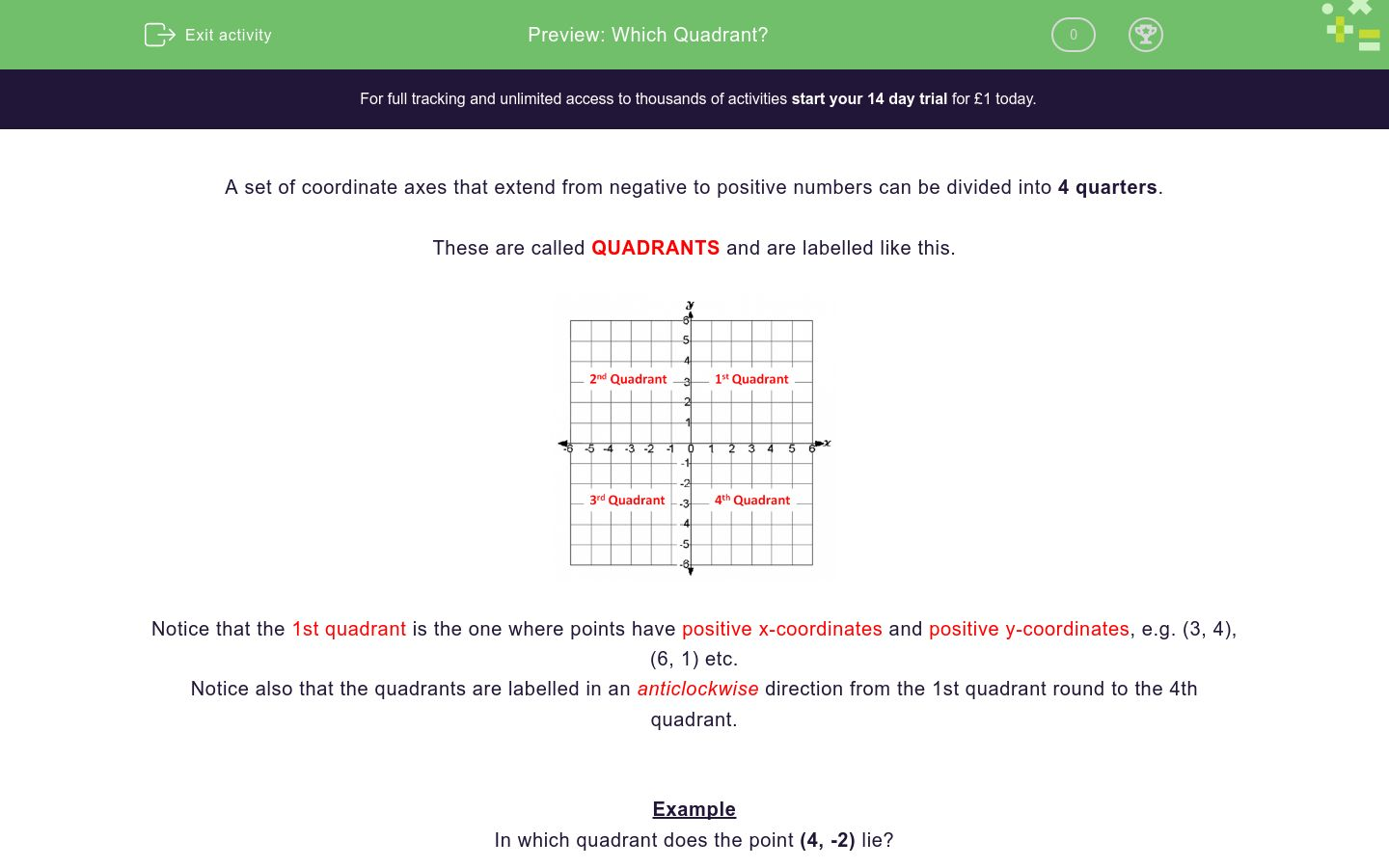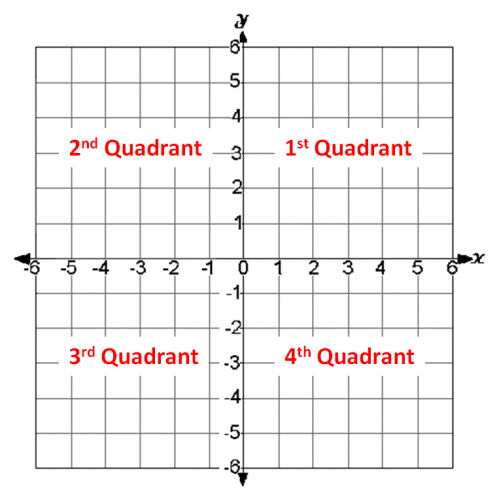### Affordable pricing from £10/month

In this worksheet, students must say in which quadrant the given coordinates lie.Key stage:  KS 3

Curriculum topic:   Algebra

Curriculum subtopic:   Use Coordinates in All Four Quadrants

Difficulty level:### QUESTION 1 of 10

A set of coordinate axes that extend from negative to positive numbers can be divided into 4 quarters.

These are called QUADRANTS and are labelled like this.Notice that the 1st quadrant is the one where points have positive x-coordinates and positive y-coordinates, e.g. (3, 4), (6, 1) etc.

Notice also that the quadrants are labelled in an anticlockwise direction from the 1st quadrant round to the 4th quadrant.

Example

In which quadrant does the point (4, -2) lie?

(4, -2) has a positive x-coordinate and and a negative y-coordinate.It is in the 4th quadrant.

In which quadrant does the point (4, 2) lie?

In which quadrant does the point (-4, 2) lie?

In which quadrant does the point (4, -4) lie?

In which quadrant does the point (-4, -4) lie?

In which quadrant does the point (-2, -3) lie?

In which quadrant does the point (-1, 7) lie?

In which quadrant does the point (2, -6) lie?

In which quadrant does the point (7, 5) lie?

In which quadrant does the point (-8, -10) lie?

In which quadrant does the point (-10, 5) lie?

• Question 1

In which quadrant does the point (4, 2) lie?

EDDIE SAYS
x-coordinate is positive.
y-coordinate is positive. This means the coordinate is in the first quadrant.
• Question 2

In which quadrant does the point (-4, 2) lie?

EDDIE SAYS
x-coordinate is negative.
y-coordinate is positive. This means the coordinate is in the second quadrant.
• Question 3

In which quadrant does the point (4, -4) lie?

EDDIE SAYS
x-coordinate is positive.
y-coordinate is negative. This means the coordinate is in the fourth quadrant.
• Question 4

In which quadrant does the point (-4, -4) lie?

EDDIE SAYS
x-coordinate is negative.
y-coordinate is negative. This means the coordinate is in the third quadrant.
• Question 5

In which quadrant does the point (-2, -3) lie?

EDDIE SAYS
x-coordinate is negative.
y-coordinate is negative. This means the coordinate is in the third quadrant.
• Question 6

In which quadrant does the point (-1, 7) lie?

EDDIE SAYS
x-coordinate is negative.
y-coordinate is positive. This means the coordinate is in the second quadrant.
• Question 7

In which quadrant does the point (2, -6) lie?

EDDIE SAYS
x-coordinate is positive.
y-coordinate is negative. This means the coordinate is in the fourth quadrant.
• Question 8

In which quadrant does the point (7, 5) lie?

EDDIE SAYS
x-coordinate is positive.
y-coordinate is positive. This means the coordinate is in the first quadrant.
• Question 9

In which quadrant does the point (-8, -10) lie?

EDDIE SAYS
x-coordinate is negative.
y-coordinate is negative. This means the coordinate is in the third quadrant.
• Question 10

In which quadrant does the point (-10, 5) lie?

EDDIE SAYS
x-coordinate is negative.
y-coordinate is positive. This means the coordinate is in the second quadrant.
---- OR ----

Sign up for a £1 trial so you can track and measure your child's progress on this activity.

### What is EdPlace?

We're your National Curriculum aligned online education content provider helping each child succeed in English, maths and science from year 1 to GCSE. With an EdPlace account you’ll be able to track and measure progress, helping each child achieve their best. We build confidence and attainment by personalising each child’s learning at a level that suits them.

Get started